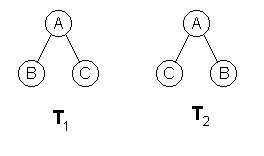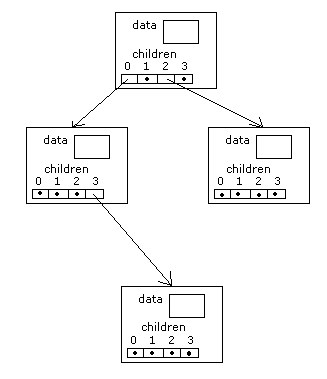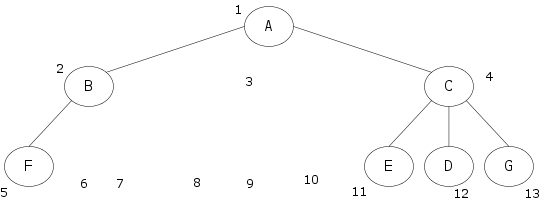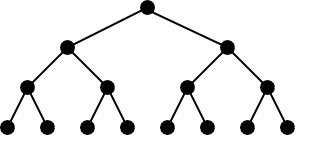# Ordered Trees

Wow, there sure are a lot of trees.

## Definition

An ordered tree is an oriented tree in which the children of a node are somehow "ordered."If T1 and T2 are ordered trees then T1 ≠ T2 else T1 = T2.

## Types of Ordered Trees

There are several types of ordered trees:

• k-ary tree
• Binomial tree
• Fibonacci tree

## Fibonoacci Trees

A Fibonacci Tree (Fk) is defined by

• F0 is the empty tree
• F1 is a tree with only one node
• Fk+2 is a node whose left subtree is a Fk+1 tree and whose right subtree is a Fk tree.
Exercise: Draw the trees F0 through F5.

## Binomial Trees

The Binomial Tree (Bk) consists of a node with k children. The first child is the root of a Bk-1 tree, the second is the root of a Bk-2 tree, etc.

Exercise: Draw the trees B0 through B5.

## k-ary Trees

A k-ary tree is a tree in which the chilren of a node appear at distinct index positions in 0..k-1. This means the maximum number of children for a node is k.

Some k-ary trees have special names

• 2-ary trees are called binary trees.
• 3-ary trees are called trinary trees or ternary trees.
• 1-ary trees are called lists.

You have to draw k-ary trees carefully. In a binary tree, if a node has one child, you can't draw a vertical line from the parent to the child. Every child in a binary tree is either a left or a right child; that must be made clear.

### Representations of k-ary Trees

Since the subtrees of a node are both indexed and bounded, we can use an array for them. Here is an example for a 4-ary tree:

```class Node {
private Object data;
private Node[] children;
.
.
.
}
```You can also use an array for the whole tree1 A 2 B 3 4 C 5 F 6 7 8 9 10 11 E 12 D 13 G

In a binary tree

• parent(i) is i / 2
• leftChild(i) is 2i
• rightChild(i) is 2i + 1

In a k-ary tree

• parent(i) is (k + i – 2) / k
• jth child of i is ki – (k–2) + j
Exercise: Prove each of these statements.

### Complete k-ary Trees

The array representation may waste a lot of space. The maximally space efficient k-ary tree is called a complete tree. A complete tree is completely filled out on every level, except perhaps on the last one, on which all we require is that all its nodes are "as far to the left as possible." Examples:Complete trees can be packed into an array with no wasted space at all.

### Perfect Trees

A perfect k-tree is a k-ary tree in which every node is either a 0-node or a k-node and all leaves are on the same level.

Perfect trees are sometimes called full trees, though some people say a full tree is just one in which every node is a 0-node or a k-node, without caring whether all leaves are on the same level or not.

### The Size of k-ary Trees

For k = 2:Level Nodes on
Level
Nodes on levels
up to and
including this one
0 1 = 20 1 = 20+1–1
1 2 = 21 3 = 21+1–1
2 4 = 22 7 = 22+1–1
3 8 = 23 15 = 23+1–1
4 16 = 24 31 = 24+1–1
h 2h 2h+1–1

For arbitrary k:

Number of nodes on level h = kh, obviously

Let S(h) = the number of nodes in a perfect binary tree of height h. Let's find a closed form.

```    S(h) = 1 + k + k2 + k3 + ... + kh

k × S(h) = k + k2 + k3 + k4 + ... + kh+1

k × S(h) - S(h) = kh+1-1

(k-1) × S(h) = kh+1-1

kh+1 - 1
S(h) = ---------
k - 1
```